Acids and Bases
Elaboration - Neutralizing Acids and Bases

From the definitions of acids and bases that we developed; operational, Arrhenius, and Brønsted-Lowry; we see that acids and bases are in many ways opposites of one another. When equal quantities of a strong acid and a strong base are mixed, or a weak acid and a weak base with similar strengths are mixed, the result is a neutral salt solution. For example, the following equation describes what happens when equal quantities of hydrochloric acid and sodium chloride are mixed: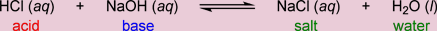To illustrate this, let us use the Virtual Laboratory Simulator to mix equal amounts of 0.02 M HCl and 0.02 M NaOH and see what we get (Figure 1)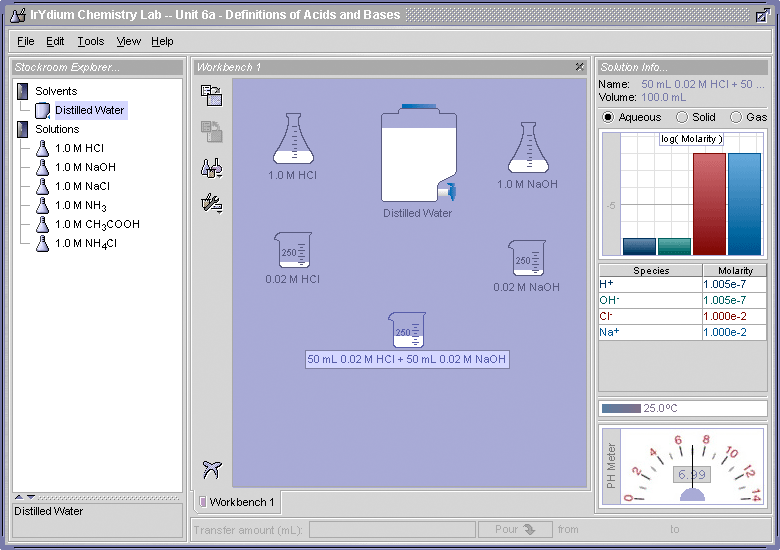If you compare the results shown in Figure 1 with those obtained in the "Try This" for Part I, Elaboration -Definitions of Acids and Bases, where a 0.01 M solution of NaCl was made, you will see that identical results were obtained.

Titration

The neutralization reaction can be used to determine the unknown concentrations of acids and bases. This is because, as a base is added to an acid to neutralize it the pH of the solution changes slowly, but at the point where the base has completely neutralized the acid, the next drop of base added will cause an abrupt increase in the pH of the solution. This abrupt change can be observed with either a pH meter or using a pH indicator such as phenolphthalein. This can be demonstrated using the Virtual Laboratory Simulator. This video tutorial for the Virtual Laboratory Simulator includes a step-by-step demonstration of how to carry out a titration using phenolphthalein as the endpoint indicator. Figure 2 shows the setup of the Virtual Laboratory Simulator that was used to monitor the pH as 0.01 M NaOH was added to 25 mL of a 0.01 M solution of HCl. A buret was used to add the NaOH to the HCl.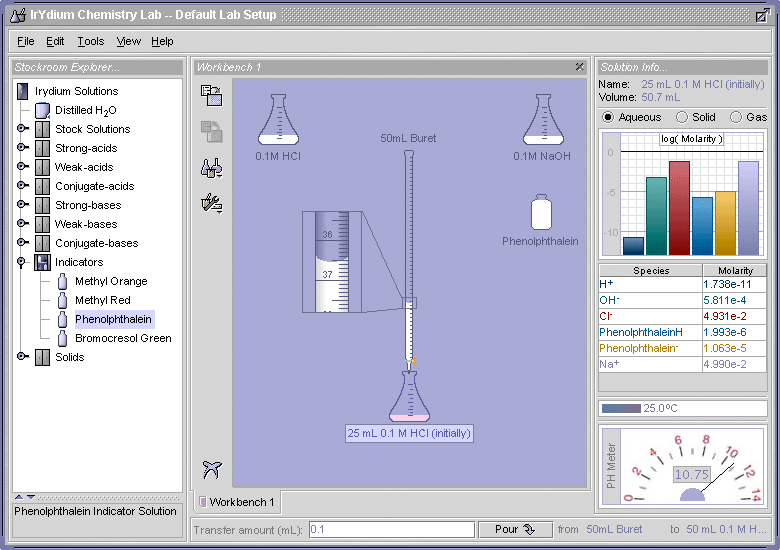During the tritration carried out as described in Figure 2, the pH was recorded after each addition of the 0.1 M NaOH. A plot of the pH versus mL of 0.1 M NaOH added is shown in Figure 3.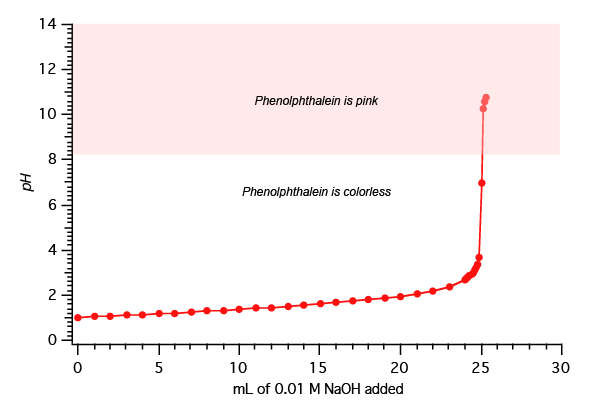In the demonstration shown above in Figures 2 and 3, the concentration of the HCl was know. If, however, it where not known, it could be determined from the volume and concentration of the base that was used to neutralize a known volume of the acid. You can then use this information to determine the concentration of the acid you had at the start of the experiment. Such an experiment used to determine an unknown concentration is called a titration. Figure 4 below shows how the unknown concentration is determined.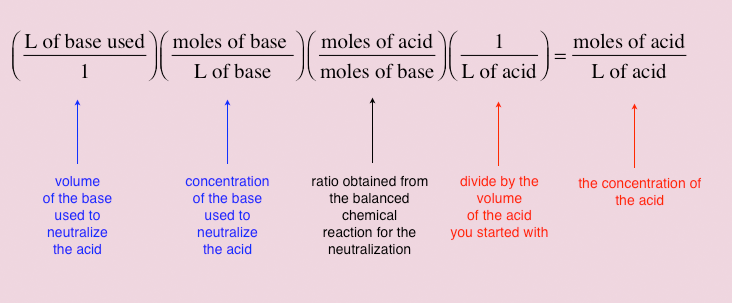For example, this is the result when we apply the calculation shown in Figure 4 to the titration shown in Figure 3: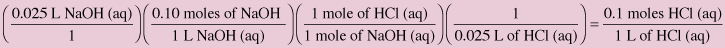Try This It requires 26.3 mL of 0.10 M KOH to titrate a 35.6 mL sample of acetic acid (CH3COOH). Calculation the initial pH of the acetic acid solution. It requires 45.0 mL of 0.20 M HCl to titrate 25.0 mL of a Ca(OH)2 solution of unknown concentration. What is the Ca(OH)2 concentration? Answer